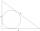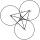Nine-gon

Calculate the perimeter of a regular nonagon (9-gon) inscribed in a circle with a radius 13 cm.

Result

x =  80.03 cm

Solution:Leave us a comment of example and its solution (i.e. if it is still somewhat unclear...):Be the first to comment!Next similar examples:

1. Circle inscribedCalculate the perimeter and area of a circle inscribed in a triangle measuring 3 , 4 and 5 cm.
2. ThalesCalculate the length of the Thales' circle described to right triangle with hypotenuse 18.4 cm.
3. Hexagon ACalculate area of regular hexagon inscribed in circle with radius r=9 cm.
4. Hexagon 5The distance of parallel sides of regular hexagonal is 61 cm. Calculate the length of the radius of the circle described to this hexagon.
5. RT - inscribed circleIn a rectangular triangle has sides lengths> a = 30cm, b = 12.5cm. The right angle is at the vertex C. Calculate the radius of the inscribed circle.
6. Height 2Calculate the height of the equilateral triangle with side 38.
7. SinesIn ▵ ABC, if sin(α)=0.5 and sin(β)=0.6 calculate sin(γ)
8. CirclesThree circles of radius 95 cm 78 cm and 64 cm is mutually tangent. What is the perimeter of the triangle whose vertices are centers of the circles?
9. n-gonWhat is the side length of the regular 5-gon inscribed in a circle of radius 12 cm?
10. n-gon IIWhat is the side length of the regular 5-gon circumscribed circle of radius 11 cm?
11. Equilateral triangleHow long should be the minimum radius of the circular plate to be cut equilateral triangle with side 19 cm from it?
12. Cable car 2Cable car rises at an angle 41° and connects the upper and lower station with an altitude difference of 1175 m. How long is the track of cable car?
13. TrigonometryIs true equality? ?
14. Theorem proveWe want to prove the sentence: If the natural number n is divisible by six, then n is divisible by three. From what assumption we started?
15. Reference angleFind the reference angle of each angle:
16. Center traverseIt is true that the middle traverse bisects the triangle?
17. 30-60-90The longer leg of a 30°-60°-90° triangle measures 5. What is the length of the shorter leg?